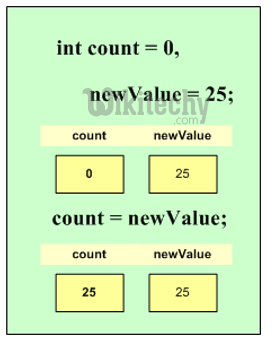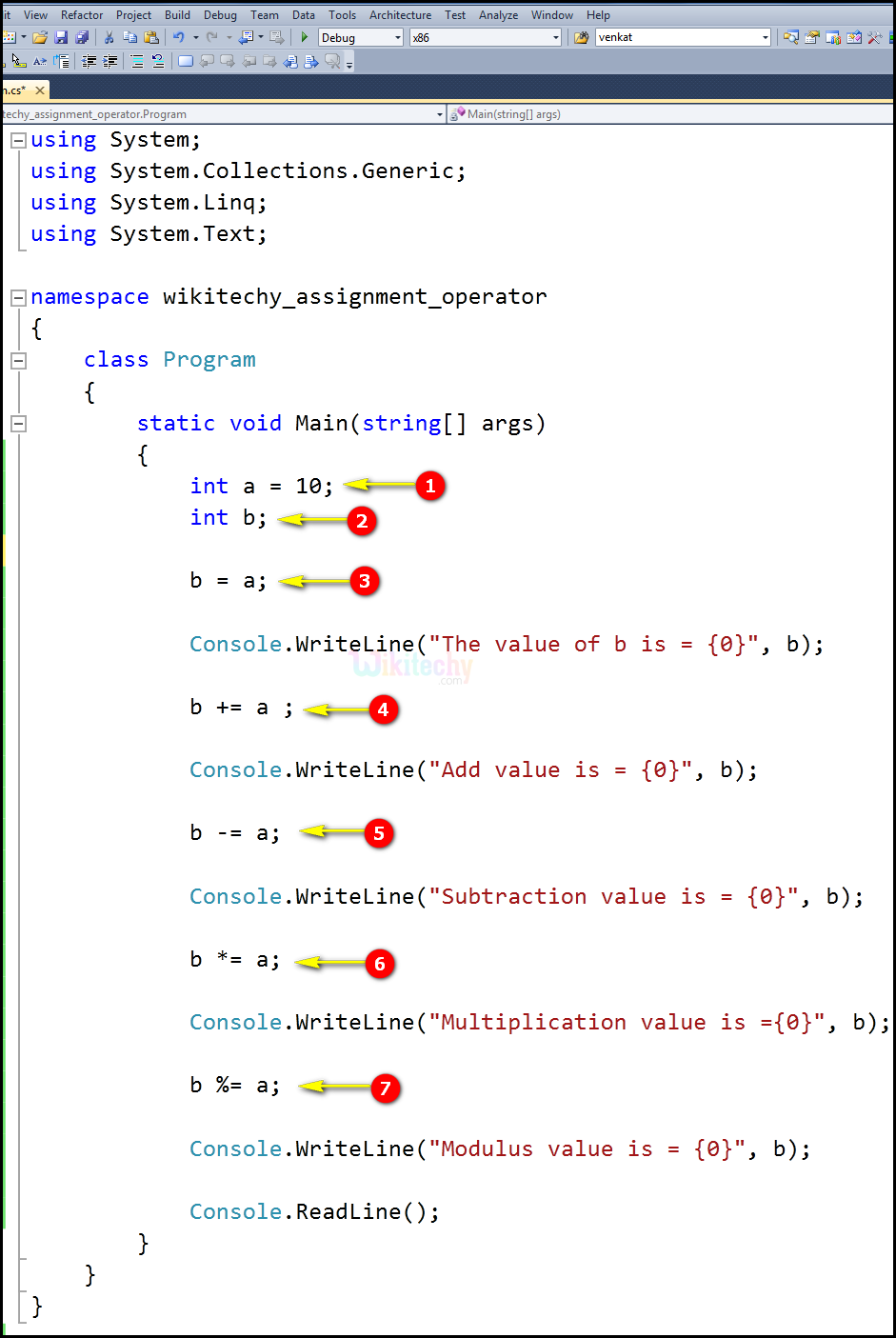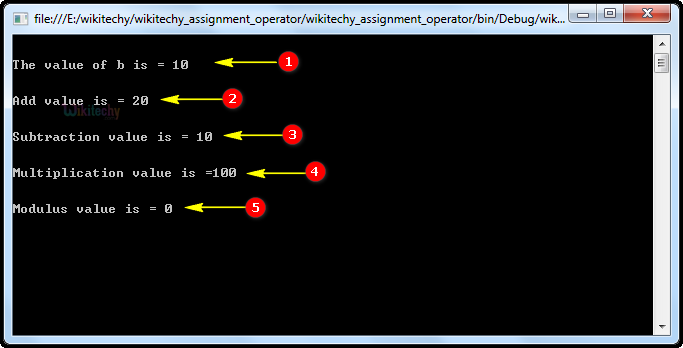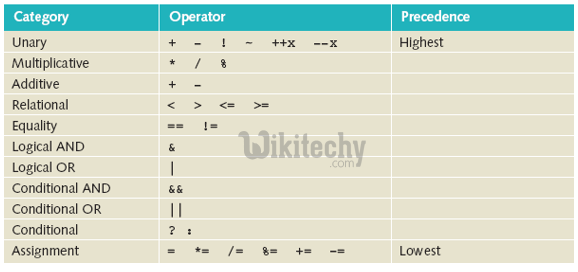# C# Assignment Operators | c# assignment - c# - c# tutorial - c# netC# Assignment Operators

## What is the use of assignment operator in C# ?

• In C# programs, values for the variables are assigned using assignment operators (=).
•• The assignment operators assign a new value to a variable, a property, an event, or an indexer element.Assignment Operator

## Code Explanation:1. int a = 10 is an integer variable value as 10.
2. int b is an integer variable.
3. In this statement we are assigning the "a" variable value 10 to "b" Variable.
4. In this statement, we are performing an addition operation that b+=a (10+10=20) and the value will be assigned for the variable "b" =20.
5. In this statement, we are performing a subtraction operation that b-=a (20-10=10) and the value will be assigned for the variable "b" =10.
6. In this statement, we are performing a multiplication operation that b*=a (10*10=100) and the value will be assigned for the variable "b" =100.
7. In this statement, we are performing a mod operation that b%=a (10%10=0) and the value will be assigned for the variable "b" =0.

## Sample C# examples - Output :1. Here in this output we have shown the assignment operation of variable "The value of b is = 10" where its output value will be displayed.
2. Here in this output we have shown the addition operation of variable "b" & "a" (10+10 =20) where its output value of the variable "Addition value is = 10" will be displayed.
3. Here in this output we have shown the subtraction operation of variable "b" & "a" (20-10=10) where its output value of the variable "Subtraction is = 10" will be displayed.
4. Here in this output we have shown the multiplication operation of variable "b" & "a" (10*10=100) where its output value of the variable "Multiplication is = 100" will be displayed.
5. Here in this output we have shown the mod operation of variable "b" & "a" (10/10=0) where its output value of the variable "Modulus is = 0" will be displayed.

## c# Operator precedence :